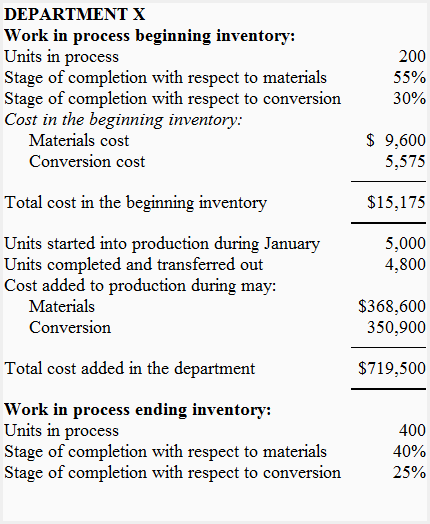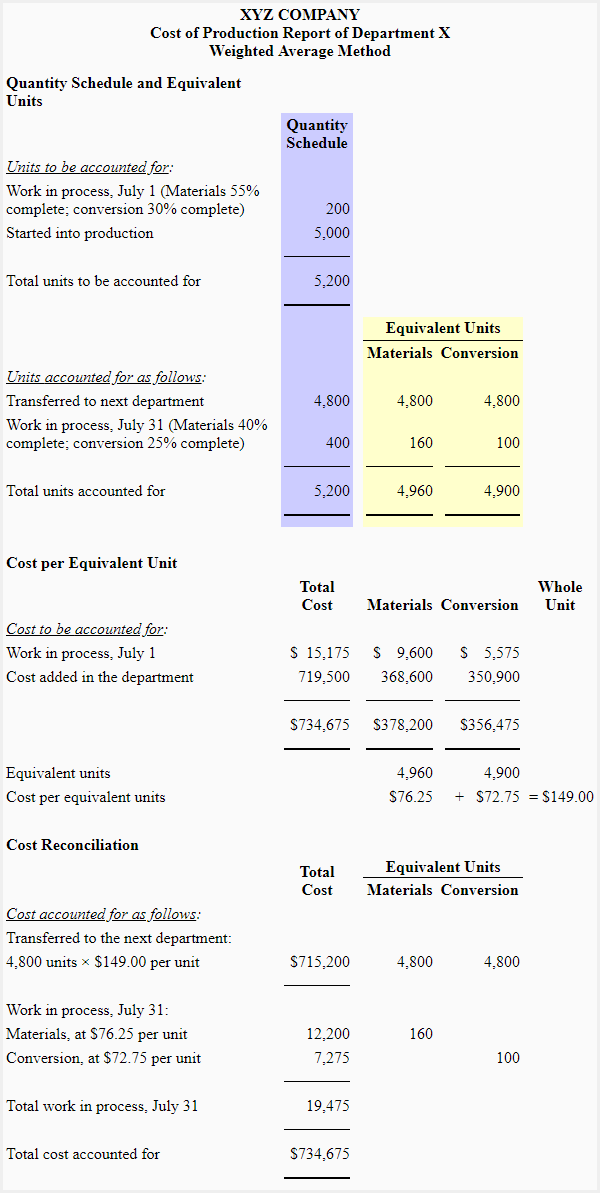# Cost of production report (CPR) – weighted average method

## Definition and explanation

The cost of production report (CPR) is a document used in process costing system that summarizes the information about the flow of units and costs through the work in process account of a processing department. It is equal to the job cost sheet prepared in a job order costing system. A separate cost of production report is prepared for each processing department.

The cost of production report is considered a key management document because it provides managers with the following crucial information about production and cost of a processing department:

1. The number of units transferred in and transferred out by a department during the month.
2. The per unit processing cost incurred by a processing department. It includes total per unit cost (i.e., total cost incurred divided by total output) as well as per unit cost for individual cost elements like direct materials, direct labor, and manufacturing overhead.
3. The highest production cost among materials, labor and manufacturing overhead.
4. The impact of a recent improvement in production process on per unit cost in a processing department.
5. A significant change in per unit cost due to unexpected change in one or more cost elements like direct materials, direct labor and manufacturing overhead.
6. The hurdles or limiting factors present in one or more processing departments that could potentially disturb the overall output efficiency of the firm.

Other names used for cost of production report are production cost report and production report.

## Sections or parts of a cost of production report

A cost of production report consists of the following three sections/parts:

1. Quantity schedule section
2. Cost per equivalent unit section
3. Cost reconciliation section

Let’s briefly describe the purpose of each section.

### 1. Quantity schedule section

Quantity schedule is the first section of a cost of production report. This section summarizes the flow of physical units through the relevant processing department and shows the equivalent units for materials and conversion costs. The percentage of completion of any units in work in process beginning and ending inventory is also shown in this section. The quantity schedule also guides in preparing the other two sections of the cost of production report.

### 2. Cost per equivalent unit section

In this section, the cost per equivalent unit is computed. Under weighted average method, it is done by dividing the total of beginning inventory cost and cost added during the period by the equivalent units of production computed under weighted average method. Click here to read how equivalent units of production are computed using weighted average method.

### 3. Cost reconciliation section

In this section, the cost charged to the department is reconciled or accounted for. Usually, the total cost charged to a department consists of the following:

• Cost of beginning work in process inventory
• Materials, labor overhead costs incurred by the department during current period
• Cost transferred in from preceding department during the period

The total of above costs is accounted for by computing the following amounts:

• Cost of units transferred to the next department or finished goods storeroom.
• Cost in ending work in process inventory.

## Example

The Jessica Company produces a product used to clean mirrors and head lights of vehicles. The company uses a process costing and has two processing departments – department X and department Y. The processing starts in department X where three different chemicals are mixed together in equal proportions. After mixing in department X, the partially completed units are transferred to department Y. The following data has been taken from department X.Required: Using above data, prepared a cost of production report of department X for the month of January.

### SolutionShow your love for us by sharing our contents.